# GED Math : Other Coordinate Geometry

## Example Questions

### Example Question #1 : Other Coordinate Geometry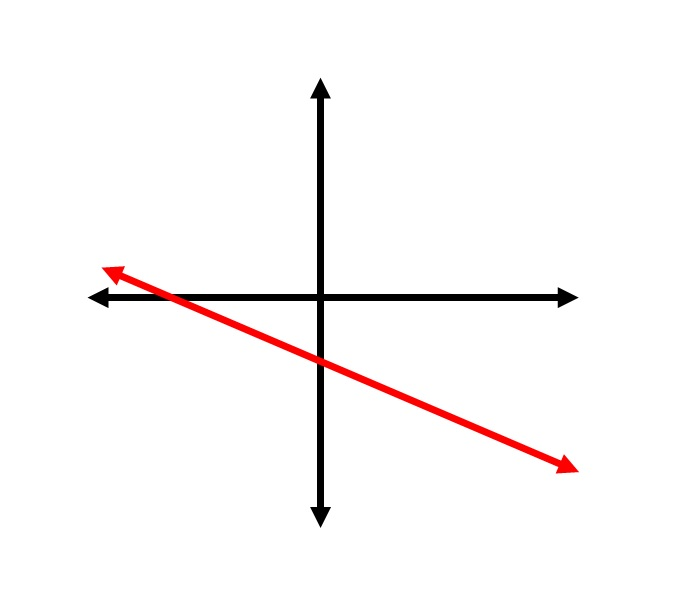Above is the graph of the equation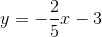.

Which of the following is the graph of the inequality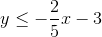?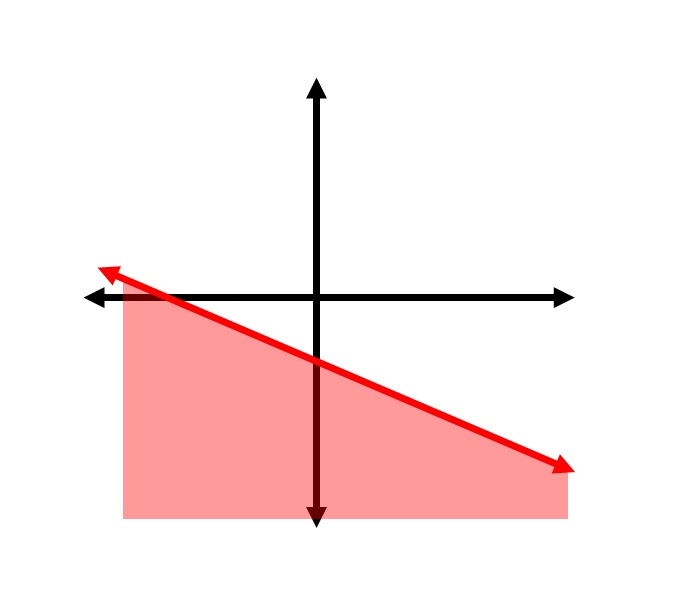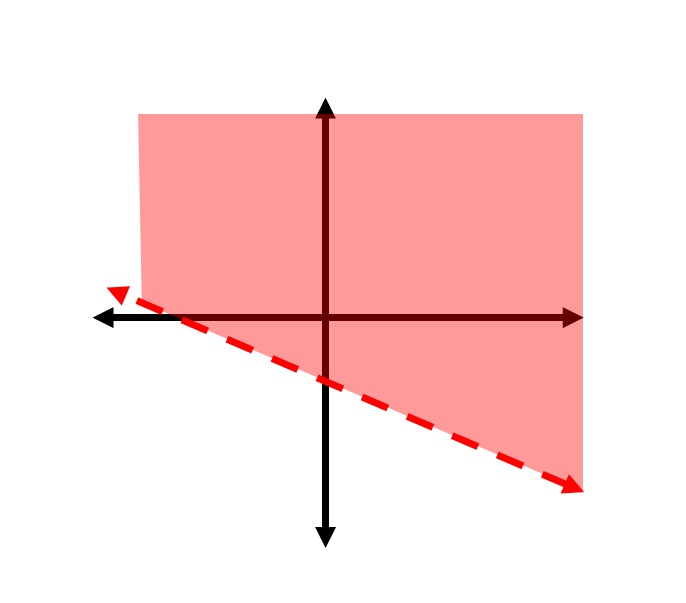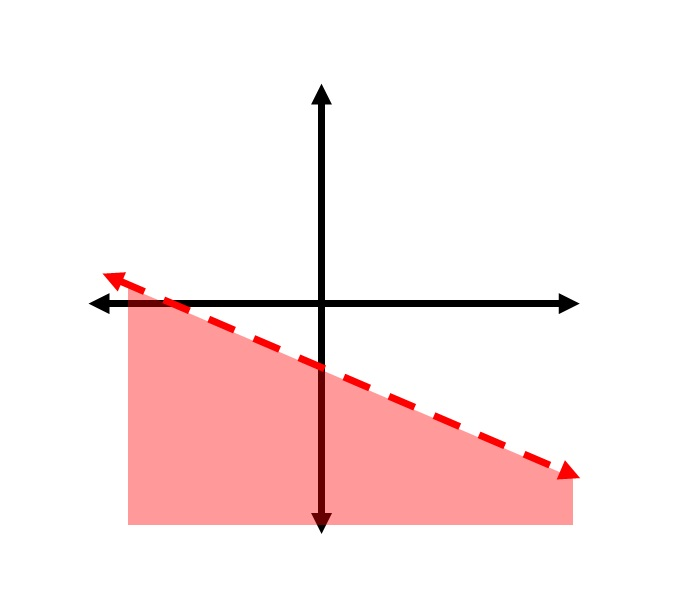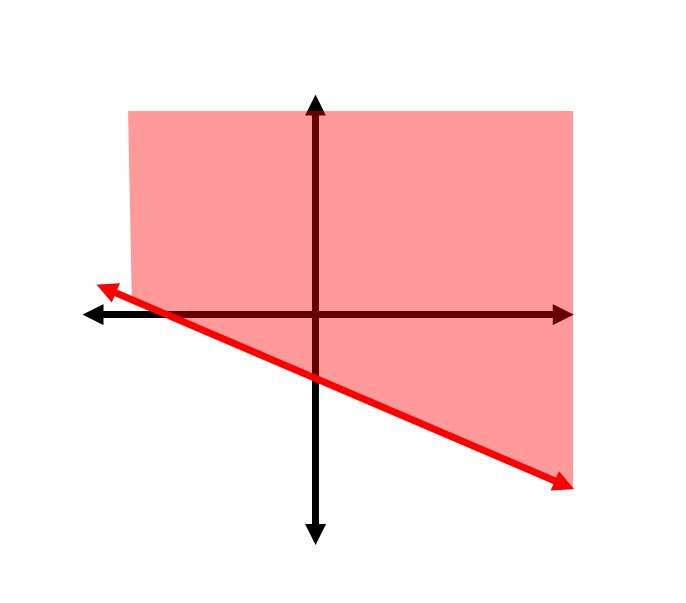Explanation:

The inequality symbol of the statementallows for the two quantities to be equal, so the line of the equationmust be part of the graph of the inequality. Therefore, we must select one of the choices with a solid line. Of the two such choices, we can determine which one to select by choosing a test point on one side of the line and substituting the coordinates in the inequality.

The easiest point to select is the origin, or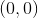.  Set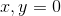in the inequality: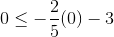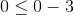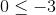This is false, so we want the choice that does not include the point. The correct choice is the graph: